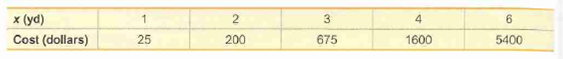Question
1 views

Topsoil The Dirt Guy Topsoil company in Durham, CT, sells (you guessed it) topsoil. It is sold by the “yard,” which is actually a cubic yard, and the variable x is the length (yd) of each side of a cube of topsoil.help_outlineImage Transcriptionclosex (yd) 4 6. Cost (dollars) 200 25 675 1600 5400 fullscreen
check_circle

Step 1

In this case, we need to construct the scatterplot for linear, quadratic, logarithmic, exponential, and power models. Also, identify mathematical model that best fits the given data.

Step 2

Step-by-step procedure to construct the scatterplot for linear model using the Excel is as follows:

• Select the column containing X and Cost.
• Choose Insert > Scatter.
• In Scatter, choose Scatter with only markers.
• Select the points in the Scatterplot and then Right click, choose Add Trendline.
• In the Trendline Options, Select Linear.
• Check Display Equation on chart and Display R-squared value on chart.

Output using the Excel is given below:

Step 3

Step-by-step procedure to construct the scatterplot for quadratic model using the Excel is as follows:

• Select the column containing X and Cost.
• Choose Insert > Scatter.
• In Scatter, choose Scatter with only markers.
• Select the points in the Scatterplot and then Right click, choose Add Trendline.
• In the Trendline Options, S...

### Want to see the full answer?

See Solution

#### Want to see this answer and more?

Solutions are written by subject experts who are available 24/7. Questions are typically answered within 1 hour.*

See Solution
*Response times may vary by subject and question.
Tagged in

### Statistics